# Show Percentages in a Stacked Column Chart in Excel

This post will guide you how to show percentages in a stacked column chart in Excel. How to display percentages as series in an Excel Stacked column chart. How to create a 100% stacked chart with percentages in Excel.

## Show Percentages in a Stacked Column Chart

Assuming that you have a list of data in the range of cells A1:D5 contain sales data for some product. And you want to create a stacked column chart with percentages based on those data. Just do the following steps:

#1 select the original data to create a stacked column chart.

#2 go to INSERT tab, click Insert Column Chart command under charts group, and select 2-D Column from the drop down list.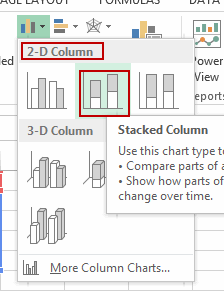#3 a stacked column chart has been created.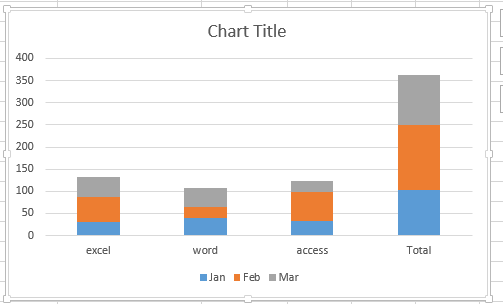#4 go to DESIGN tab, click Switch Row/Column command under Data group.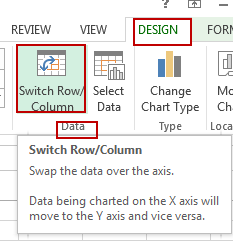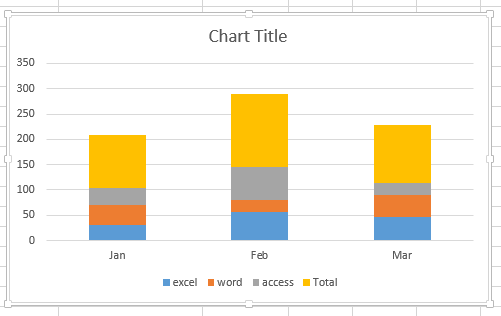#5 go to Layout tab, click Add Chart Element command under Chart Layouts group. And then click Data Labels, select Center from the popup menu list.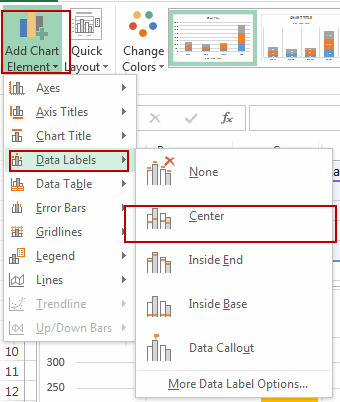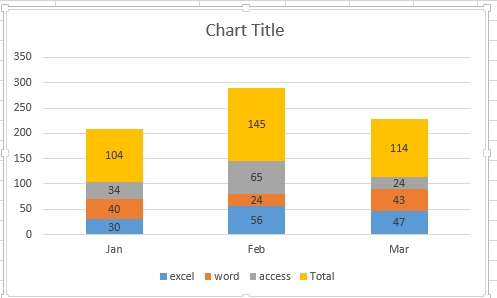x
How to Select Every Other Row in Excel

#6 select one blank range and copy all product names into it.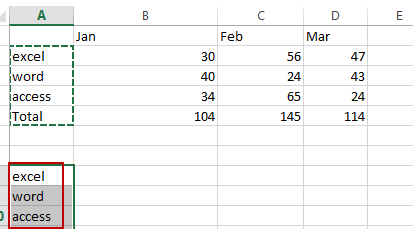#7 you need to divide each value in the table above by the total value in the same row, so you can type the following formula into the formula box of cell B8 and then drag the fill handle over other cells to calculate the percentage values.

`=B2/B\$5`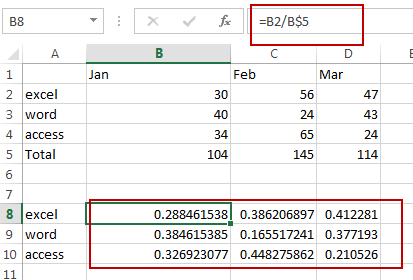#8 select those decimal numbers, and then go to HOME tab, click Number format drop down list under Number group. Or directly click % icon.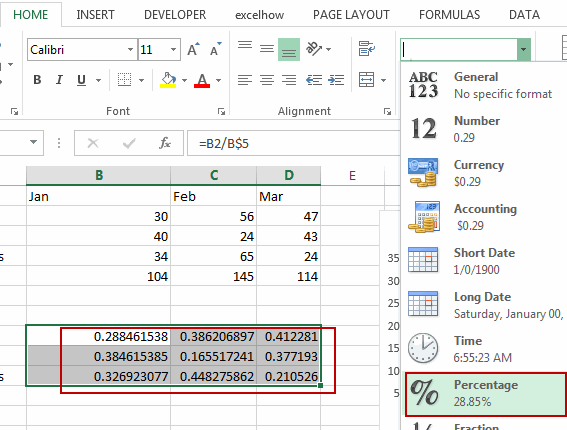All selected numbers have been changed to percentage format.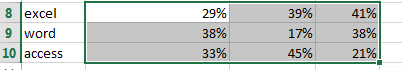#9 select one data label in the stacked column chart, and then type = in the formula bar, and then select percentage value, and press Enter key.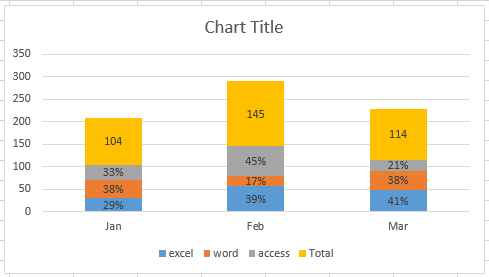You will see that the data label has been displayed as percentage. You need to repeat this step to change other data labels.

Related Posts

If Cell is This Value or That Value

IF function is frequently used in Excel worksheet to return you expect “true value” or “false value” based on the result of logical test. If you want to see if a cell is A or B, and if one of ...

If Value is Greater Than A Certain Value

IF function is frequently used in Excel worksheet to return you expect “true value” or “false value” based on the logical test result. If you want to see if a value in one cell is greater than a specific value, ...

If Cell is Not Blank

IF function is frequently used in Excel worksheet to return you expect “true value” or “false value” based on the result of created logical test. If you want to see if a cell is blank or not, and leave some ...

VBA Macro For VLOOKUP From Another Sheet

In the previous post, you should know that how to fix or remove the #N/A error when using VLOOKUP formula to lookup value from another sheet. And this post will show you how to use VBA code to vlookup data ...

If Cell is Blank

IF function is frequently used in Excel worksheet to return you expect “true value” or “false value” based on the result of created logical test. If you want to see if a cell is blank or not, and leave some ...

If Cell Equals Certain Text String

IF function is frequently used in Excel worksheet to return you expect “true value” or “false value” based on the result of created logical test. If you want to see if cell equals a certain text string like “Win”, you ...

If Cell Contains Either Text1 or Text2

IF function is frequently used in Excel worksheet to return “true value” or “false value” based on the logical test result. If you want to see if cell contains certain substring1 like “abc” or substring2 like “def”, and returns true ...

If Cell Contains Certain Text OR Equals Certain Text

IF cell equals certain text IF function is frequently used in Excel worksheet to return “true value” or “false value” based on the logical test result. If you want to test values to see if they equal certain text like ...

VLOOKUP From Another Sheet Not Working

In the previous post, you should know that how to fix or remove the #N/A error when using VLOOKUP formula to lookup value from another sheet. And this post will show you reasons why your VLOOKUP formula is not working ...

If Cell Begins with One of Three Supplied Characters

If you want to test values to see if they begin with some given specific characters like “x”, ”y”, or “z”, you can create a formula with COUNTIF and SUM functions to return results. EXAMPLE You can see “TRUE” or ...

Sidebar